## Logical Block Addressing

This entry is a continuation of my attempt to write a functioning IBM PC bootloader. Find the previous entry of the series here and the first entry here.

Turns out disks are difficult. For one thing, they have two sides. But they also have tracks and sectors.

If I want to talk to a disk, I have to tell it which cylinder I want, which head (side, on a floppy disk) and which sector (of that head of the cylinder). But an operating system deals with possibly different disk formats and file systems report file locations based on logical blocks, which are sectors (or clusters of sectors, to make it more interesting) numbered in logical order, independent of the physical disk, from 0 to whatever is the last block.

The conversion algorithm is relatively straightforward. You need the number of sectors per track of your disk (stored in bootsector), the number of heads per cylinder (stored in bootsector, hint: on a floppy disk it's 2), and a distant but retrievable memory of the fact that sectors are one-based but heads and tracks are zero-based, and two years of Torah study. (I.e. the very first block of the disk is the first sector of the first track of the first head which is sector 1 of track 0 of head 0. The two years of Torah study are not strictly necessary.)

Here is the conversion from cylinder-head-sector to logical block in PowerShell:

\$block = (\$cylinder * \$heads + \$head) * \$sectors + (\$sector - 1)

\$heads is the number of heads per cylinder, \$sectors is the number of sectors per track.

And here is the conversion from logical block to cylinder-head-sector in PowerShell:

\$cylinder = [Math]::Floor(\$block / (\$heads * \$sectors))

\$head = ([Math]::Floor(\$block / \$sectors)) % \$heads

\$sector = (\$block % \$sectors) + 1

The % operator is modulo. The [Math]::Floor is needed to convince PowerShell to do an integer division. My implementation of the algorithm appears to generate the same results as this Web application someone wrote. Remember that a 1.44 MB floppy disk formatted for IBM PC has 2 heads (i.e. 2 sides) and 18 sectors per track. (It also has a number of cylinders and hence tracks but that's not very important for this exercise.)

The bootsector of the disk tells the observant disk reader the number of heads per cylinder (sides per cylinder in the case of a normal floppy disk) and the number of sectors per track.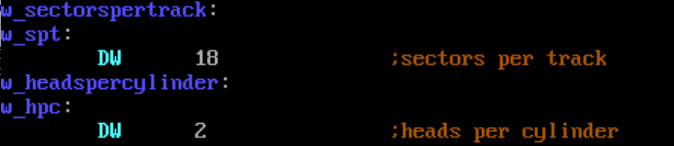Note that I have those entries two labels, one that is explanatory and one that fits better in a line of code.

In the code I have prepared, just after the example file name, four words to store the logical block number, the cylinder number, the head number and the sector number. (They are stored just behind the file name so I can actually find the four numbers in a memory dump.)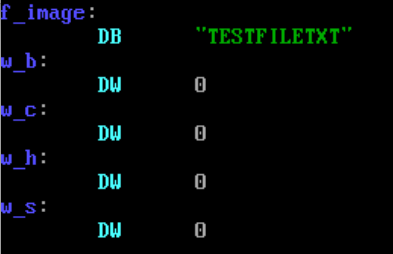The assembler implementation of the lba2chs and chs2lba algorithms hence refer to both the bootsector constants and those four fixed memory locations for input and output.

This is the assembler implementation of chs2lba.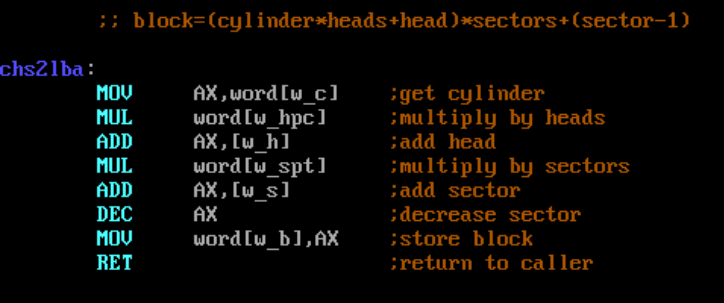Note that it gets cylinder, head and sector from w_c, w_h, and w_s respectively. It finally writes the logical block number into w_b.

And this is the assembler implementation of lba2chs.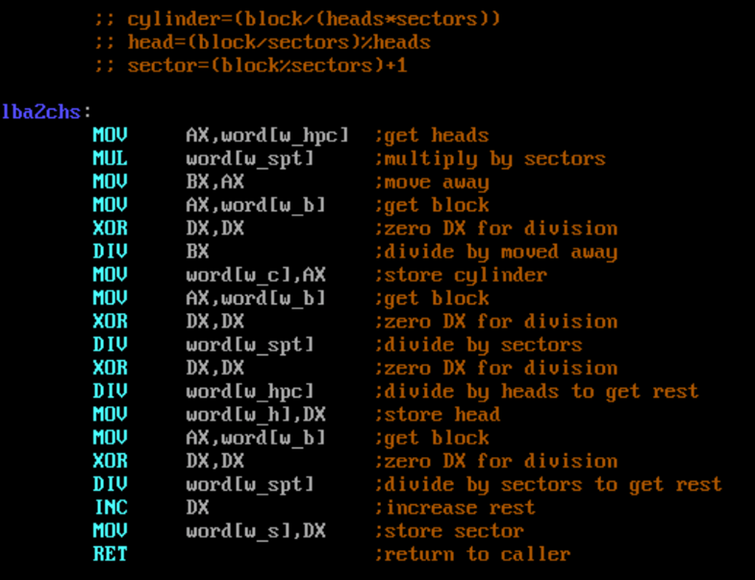Note that it gets the logical block number from w_b and ultimately writes cylinder, head, and sector to w_c, w_h, and w_s.

Also note that Intel's integer division for 16 bit values works by dividing DX:AX by the given divisor, putting the result into AX and the rest into DX. That means that both DX and AX will be destroyed in the process and, and I cannot stress this enough, DX should be zero before attempting the division if the dividend is supposed to be in AX.

This is a test with cylinder 2, head 0, and sector 7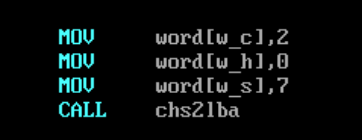The PowerShell implementation claims that this is logical block 78:

.\chs2lba.ps1 2 0 7

78

(Note that 78 in decimal is 0x4E in hexadecimal.)

The result of the assembler version of the code is this: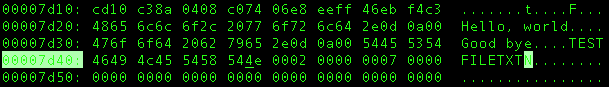Note that four words (16 bit values) following TESTFILETXT (i.e. where the cursor is):

4e00 (the output logical block, lower byte first)
0200 (the input cylinder, lower byte first)
0000 (the input head, lower byte first)
0700 (the input sector, lower byte first)

So this appears to work. c-h-s 2-0-7 is lba 0x4E (or 78 in decimal).

The other way around: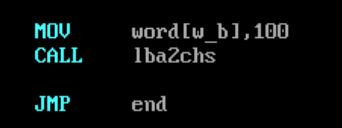Result per PowerShell is

.\lba2chs.ps1 100
2
1
11

(Note that 100 is a decimal number and translates to 0x64 in hexadecimal. 11 is also decimal and translates to 0x0B.)

And the assembler result: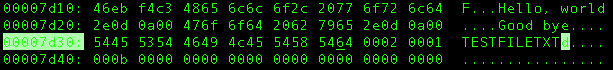Where the cursor is:

6400 (the input logical block, lower byte first)
0200 (the output cylinder, lower byte first)
0100 (the output cylinder, lower byte first)
0b00 (the output sector, lower byte first)

So apparently lba 0x64 is c-h-s 2-1-0xB. Now that's good, isn't it?

To be continued...

© Andrew Brehm 2016